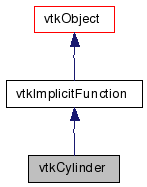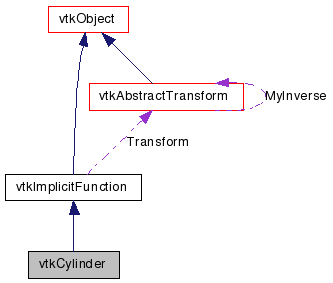# vtkCylinder Class Reference

`#include <vtkCylinder.h>`

Inheritance diagram for vtkCylinder:[legend]
Collaboration diagram for vtkCylinder:[legend]

## Detailed Description

implicit function for a cylinder

vtkCylinder computes the implicit function and function gradient for a cylinder. vtkCylinder is a concrete implementation of vtkImplicitFunction. Cylinder is centered at Center and axes of rotation is along the y-axis. (Use the superclass' vtkImplicitFunction transformation matrix if necessary to reposition.)

Warning:
The cylinder is infinite in extent. To truncate the cylinder use the vtkImplicitBoolean in combination with clipping planes.
Tests:
vtkCylinder (Tests)

Definition at line 37 of file vtkCylinder.h.

virtual void SetCenter (double, double, double)
virtual void SetCenter (double)
virtual double * GetCenter ()
virtual void GetCenter (double data)
vtkCylinder ()
~vtkCylinder ()

## Public Types

typedef vtkImplicitFunction Superclass

## Public Member Functions

virtual const char * GetClassName ()
virtual int IsA (const char *type)
void PrintSelf (ostream &os, vtkIndent indent)
void EvaluateGradient (double x, double g)
double EvaluateFunction (double x)
double EvaluateFunction (double x, double y, double z)

## Static Public Member Functions

static int IsTypeOf (const char *type)
static vtkCylinderSafeDownCast (vtkObject *o)
static vtkCylinderNew ()

## Protected Attributes

double Center 

## Member Typedef Documentation

Reimplemented from vtkImplicitFunction.

Definition at line 40 of file vtkCylinder.h.

## Constructor & Destructor Documentation

 vtkCylinder::vtkCylinder ( ) ` [protected]`

Set/Get cylinder center

 vtkCylinder::~vtkCylinder ( ) ` [inline, protected]`

Set/Get cylinder center

Definition at line 68 of file vtkCylinder.h.

## Member Function Documentation

 virtual const char* vtkCylinder::GetClassName ( ) ` [virtual]`

Reimplemented from vtkImplicitFunction.

 static int vtkCylinder::IsTypeOf ( const char * name ) ` [static]`

Return 1 if this class type is the same type of (or a subclass of) the named class. Returns 0 otherwise. This method works in combination with vtkTypeMacro found in vtkSetGet.h.

Reimplemented from vtkImplicitFunction.

 virtual int vtkCylinder::IsA ( const char * name ) ` [virtual]`

Return 1 if this class is the same type of (or a subclass of) the named class. Returns 0 otherwise. This method works in combination with vtkTypeMacro found in vtkSetGet.h.

Reimplemented from vtkImplicitFunction.

 static vtkCylinder* vtkCylinder::SafeDownCast ( vtkObject * o ) ` [static]`

Reimplemented from vtkImplicitFunction.

 void vtkCylinder::PrintSelf ( ostream & os, vtkIndent indent ) ` [virtual]`

Methods invoked by print to print information about the object including superclasses. Typically not called by the user (use Print() instead) but used in the hierarchical print process to combine the output of several classes.

Reimplemented from vtkImplicitFunction.

 static vtkCylinder* vtkCylinder::New ( ) ` [static]`

Reimplemented from vtkObject.

 double vtkCylinder::EvaluateFunction ( double x ) ` [virtual]`

Evaluate cylinder equation F(x,y,z) = (x-x0)^2 + (z-z0)^2 - R^2.

Implements vtkImplicitFunction.

 double vtkCylinder::EvaluateFunction ( double x, double y, double z ) ` [inline]`

Evaluate cylinder equation F(x,y,z) = (x-x0)^2 + (z-z0)^2 - R^2.

Reimplemented from vtkImplicitFunction.

Definition at line 49 of file vtkCylinder.h.

 void vtkCylinder::EvaluateGradient ( double x, double g ) ` [virtual]`

Implements vtkImplicitFunction.

 virtual void vtkCylinder::SetRadius ( double ) ` [virtual]`

 virtual double vtkCylinder::GetRadius ( ) ` [virtual]`

 virtual void vtkCylinder::SetCenter ( double , double , double ) ` [virtual]`

Set/Get cylinder center

 virtual void vtkCylinder::SetCenter ( double  ) ` [virtual]`

Set/Get cylinder center

 virtual double* vtkCylinder::GetCenter ( ) ` [virtual]`

Set/Get cylinder center

 virtual void vtkCylinder::GetCenter ( double data ) ` [virtual]`

Set/Get cylinder center

## Member Data Documentation

 double vtkCylinder::Radius` [protected]`

Definition at line 68 of file vtkCylinder.h.

 double vtkCylinder::Center` [protected]`

Definition at line 72 of file vtkCylinder.h.

The documentation for this class was generated from the following file:

Generated on Wed Aug 24 11:32:48 2011 for VTK by1.5.6